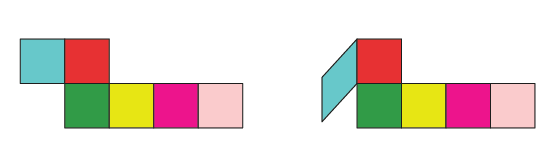# Class 7 Math NCERT SOLUTIONS FOR Chapter – 15 Visualizing Solid Shapes ex – 15.1

## Visualizing Solid Shapes

1. Identify the nets which can be used to make cubes (cut out copies of the nets and try it):

(i)

Solution:-

The given net cannot be folded as cube.

Because, it can be folded as below,(ii)

Solution:-

The given net can be folded as cube.

Because, it can be folded as below,

(iii)

Solution:-

The given net can be folded as cube.

Because, it can be folded as below,

(iv)

Solution:-

The given net can be folded as cube.

Because, it can be folded as below,

(v)

Solution:-

The given net cannot be folded as cube.

Because, it can be folded as below,

(vi)

Solution:-

The given net can be folded as cube.

Because, it can be folded as below,

2. Dice are cubes with dots on each face. Opposite faces of a die always have a total of seven dots on them.

Here are two nets to make dice (cubes); the numbers inserted in each square indicate the number of dots in that box.

Insert suitable numbers in the blanks, remembering that the number on the opposite faces should total to 7.

Solution:-

(i)

By observing the above figure the sum of the opposite faces is equal to 7.

So, the given net can be folded into a net of dice.

(ii)

By observing the above figure the sum of the opposite faces is equal to 7.

So, the given net can be folded into a net of dice.

3. Can this be a net for a die?

Solution:-

The given net is folded as dice is shown in figure below,

No, this cannot be a net for a die.

By observing the figure we can say that, one pair of opposite face will have 1 and 4, another pair of opposite face will have 3 and 6 the sum of these two opposite faces are not equal to 7.

4. Here is an incomplete net for making a cube. Complete it in at least two different ways. Remember that a cube has six faces. How many are there in the net here?

(Give two separate diagrams. If you like, you may use a squared sheet for easy manipulation.)

Solution:-

In the given net there are 3 faces and it can be completed as shown below,

5. Match the nets with appropriate solids:

Solution:-

(a) (ii)

Because, the net (ii) can be folded as square.

(b) (iii)

Because, the net (iii) can be folded as cylinder.

(c) (iv)

Because, the net (iv) can be folded as cone.

(d) (i)

Because, the net (i) can be folded as pyramid.